Computer Science

# Lab 2a - If

## Preparation

Reminder, you should work in teams of 2 or 3 when solving the lab problems. Learning to code together is an important part of this class.

### 1 minute

Download the zip file for this lab, located above. This zip file has worlds that you will use for Bit. Extract the files and put them in your `cs110` directory in a folder called `lab2a`.

## Exercise 1

### 5 minutes

Bit starts in this world:(a) Can you figure out what this code does?

On a piece of scratch paper, draw out what you think the final world will look like.

``````from byubit import Bit

def turn_or_change(bit):
if bit.is_red():
bit.left()
elif bit.is_green():
bit.paint('blue')
elif bit.is_blue():
bit.paint('green')

@Bit.worlds('exercise1')
def go(bit):
while bit.front_clear():
bit.move()
turn_or_change(bit)

if __name__ == '__main__':
go(Bit.new_bit)
``````

(b) Run the code

Inside of `lab2a`, run the file called `exercise1.py` and check your answer.

## Exercise 2

### 5 minutes

Bit starts in this world: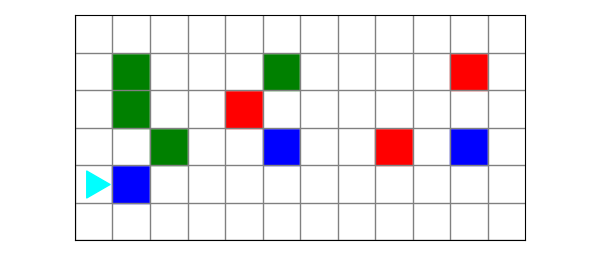(a) Can you figure out what this code does?

On a piece of scratch paper, draw out where you think Bit will end up. Show which square and which direction Bit will face.

``````from byubit import Bit

def follow_rules(bit):
if bit.is_blue():
bit.left()
elif bit.is_green():
bit.right()
elif bit.is_red():
bit.right()
bit.right()

@Bit.worlds('exercise2')
def go(bit):
while bit.front_clear():
bit.move()
follow_rules(bit)

if __name__ == '__main__':
go(Bit.new_bit)

``````

(b) Run the code

Inside of `lab2a`, run the file called `exercise2.py` and check your answer.

## Fix reds

### 15 minutes

For this problem, Bit starts in this world: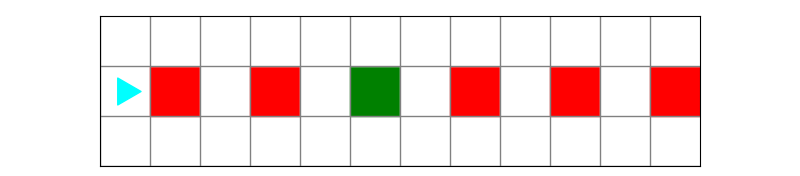Bit needs to move to the green square, and then turn all the red squares to blue: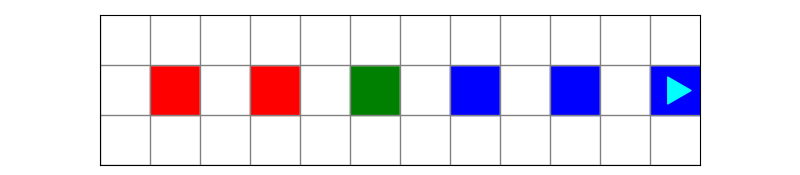(1) Draw the problem out with a friend. Use a drawing or a flow chart.

(2) Write the code with a friend. Use decomposition — break the problem up into multiple functions, each one doing a small thing.

(3) Come together as a class and discuss the solution.

## Flowers

### 15 minutes

For this problem, Bit wants to grow some flowers. Bit starts in this world: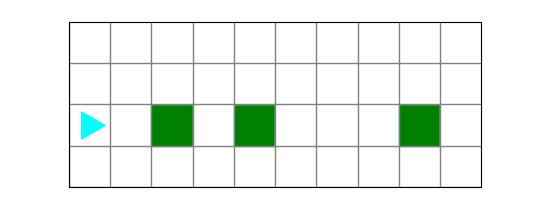Each of the green squares represents a flower stem. Move Bit to each flower stem and grow a flower on top of it: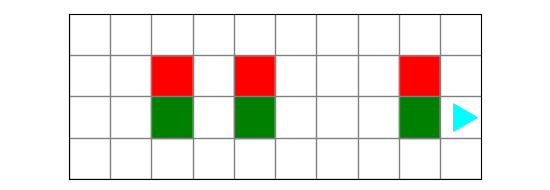(1) Draw the problem out with a friend. Use a drawing or a flow chart.

(2) Write the code with a friend. Use decomposition — break the problem up into multiple functions, each one doing a small thing.

(3) Come together as a class and discuss the solution.

## Wrapup

### 5 minutes

Discuss any questions about if statements